# (Elementary) Vector Spaces

• Highway
I think I have the first one now!In summary, the student is struggling with proofs and trying to figure out how to begin. They are also confused about the vector space concept and don't know how to show that V is not a vector space.

## Homework Statement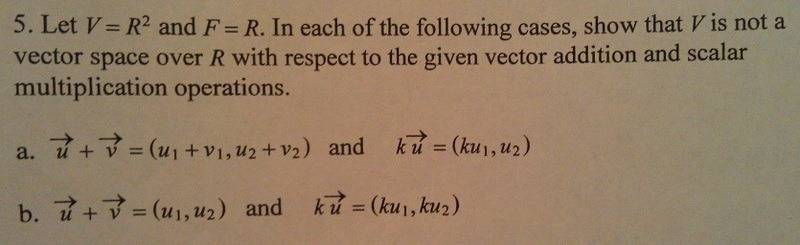## Homework Equations

addition / multiplication -- showing that the vectors (in this case) aren't closed under addition and scalar multiplication. . .

## The Attempt at a Solution

I really get stuck with proofs: how to begin / where to go / etc.

I know that I am supposed to show that this isn't a vector space by it not being closed under addition and/or scalar multiplication -- but I don't know how to do that.

The examples in the book are of polynomials -- so they take u = a2x2+ a1x + a0 and add it to v = b2x2+ b1x + b0 then separate like terms, and show that it is closed under addition. I don't know how to do something like this here.

Also, I don't know if the scalar multiplication (for part a) is a typo or not). . .

Other thoughts:

for part a), is it possible that the vector addition holds, but due to the ku = (ku1, u2) not following the law of scalar multiplication, i.e. ku = (ku1, ku2, ... , kun) that it isn't closed?

for part b), there are no v elements in the final solution, implying that v is the 0 (zero vector), and due to part of the definition, that does not allow it to be closed under these previous properties?

I know the concepts of what we are learning / supposed to be showing|doing, but I don't know how to do it :P

Well, you probably have a list of axioms that have to be satisfied in order for a set of vectors to be a vector space. So, in order to show that V is not a vector space with the given addition and multiplication, you have to find at least one of the axioms that isn't satisfied. It seems that you are a bit confused about what they're asking, because you're assuming that you have a specific way of defining addition and scalar multiplication. In other words, based on your second post, it sounds like you're assuming that when you have two vectors, that there is only one way to add them and there is only one way that scalar multiplication can work.

But for this exercise, they're defining addition and scalar multiplication for you, and they're asking you to find which vector space axiom(s) are not satisfied with these definitions of addition and multiplication. It might help to try to pretend that you've never learned how to add vectors or do scalar multiplication. Verify each of the axioms until you come across a problem. For instance, is it true that for all x and y, x+y = y+x under the definition of vector addition in part a? And so on.

I understand what you're saying, but I still don't have the first clue of how to go about doing this. . . sorry :\

While not an "axiom" itself, it can be shown from the axioms that 0v= 0. That is, that the scalar, 0, times any vector gives the 0 (additive identity) vector. Is that true here?

Last edited by a moderator:
$\mathbb R^2$ is clearly closed under these operations. To say that it's not, is to say that the right-hand side of at least one of the equalities in the picture is not a member of $\mathbb R^2$.

What you need to do is to check which ones of the vector space axioms are satisfied. For example, is it true that for all $\vec u,\vec v\in\mathbb R^2$, $\vec u+\vec v=\vec v+\vec u$? This is how you check that in part a:

Let $\vec u,\vec v\in\mathbb R^2$ be arbitrary. We have $$\vec u+\vec v=(u_1,u_2)+(v_1,v_2)=(u_1+v_1,u_2+v_2)=(v_1+u_1,v_2+u_2)=\vec v+\vec u.$$ So the answer is "yes", that axiom is satisfied in problem a. Now keep checking axioms until you find one that isn't satisfied.

thanks guys! this all helped a lot, i think i got the first one (it doesn't pass distributivity), so i will keep you posted about the second one!

Hmmm... don't want to disappoint you, Highway, but I think the first one does satisfy distributivity (I could be wrong, though). Could you explain how you got that it does satisfy distributivity?

there are 2 distributive laws:

k(u+v) = ku + kv

and:

(k+m)u = ku + mu.

one of these is not satisfied.

thanks for double checking this for me, i really appreciate it!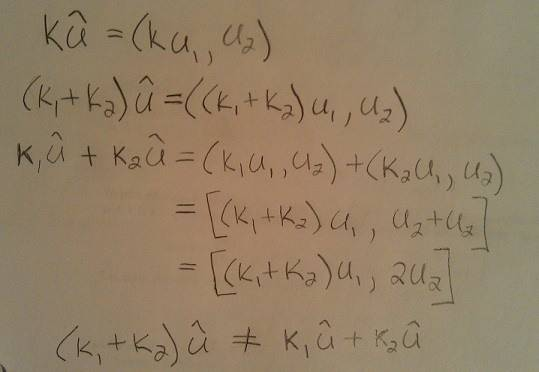Ah, looks good! I think I was wrong. The way I approached it was like this: I think one of the axioms is that 0 times x has to be the zero vector for all x. But if x is not the zero vector, then 0x turns out to be (0, x_2), which is not the zero vector. I'm not sure if that was one of the axioms. But I think the way you did it is right.

murmillo said:
Ah, looks good! I think I was wrong. The way I approached it was like this: I think one of the axioms is that 0 times x has to be the zero vector for all x. But if x is not the zero vector, then 0x turns out to be (0, x_2), which is not the zero vector. I'm not sure if that was one of the axioms. But I think the way you did it is right.

that is def one of the axioms, but this is for part 5a) not 5b) -- you might want to look over each of them, because i think the zero vector axiom you're talking about applies for 5b.

i don't get what you're saying...that axiom must be satisfied in order for you to have a vector space for 5a) and since it isn't satisfied, the V in 5a) isn't a vector space.

murmillo said:
i don't get what you're saying...that axiom must be satisfied in order for you to have a vector space for 5a) and since it isn't satisfied, the V in 5a) isn't a vector space.

do i have to show that as well, or can i "stop here" since i already found one axiom that isn't satisified? (for 5a)

you can stop once you've found one axiom that doesn't work (which you did). i was just giving another way of looking at it...

murmillo said:
you can stop once you've found one axiom that doesn't work (which you did). i was just giving another way of looking at it...

ok thanks. . .

is this ok so far for 5b? I am kinda stuck at this point and don't know where to go from here -- assuming that it is correct. . .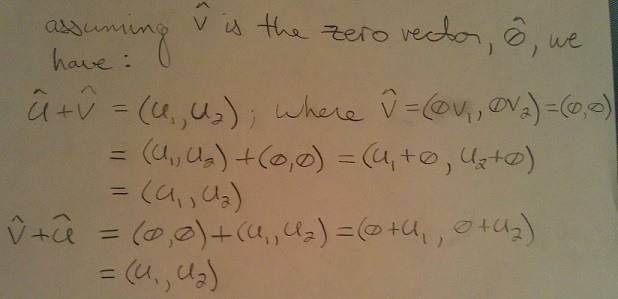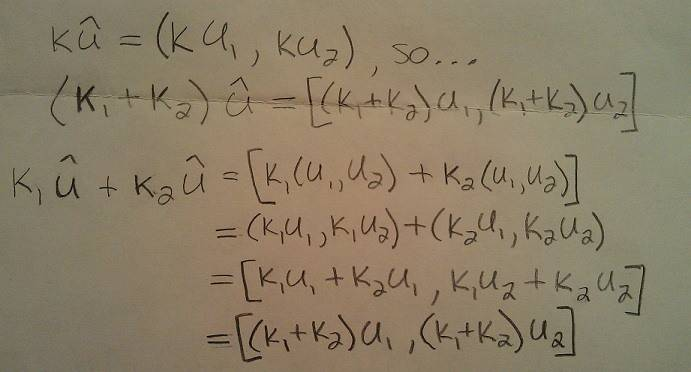part 5b):
When you do these calculations, you have to do addition the way they defined it.
The way they defined addition is
(u1, u2) + (v1, v2) = (u1, u2).
But when you did addition, you did
(u1, u2) + (v1, v2) = (u1 + v1, u2+v2), which you are not allowed to do.
So, what is (0, 0) + (x1, x2) when (x1, x2) is any vector in V?

murmillo said:
part 5b):
When you do these calculations, you have to do addition the way they defined it.
The way they defined addition is
(u1, u2) + (v1, v2) = (u1, u2).
But when you did addition, you did
(u1, u2) + (v1, v2) = (u1 + v1, u2+v2), which you are not allowed to do.
So, what is (0, 0) + (x1, x2) when (x1, x2) is any vector in V?

im not following where this is going though, i guess i don't see why your way is correct, even though i do now understand that i have to do the addition in the way that it is defined. . .

hmm, is it ok to say that v = (0,0) or does it already say that in the definition of the problem statement?

sorry for being so new at this. . .

I see the problem. So, the way they defined addition in 5b is: the sum of any two vectors is the first vector. Always. That's the important part that I think you are not getting.

By the way, don't worry if you're finding this difficult. I struggled too when I took linear algebra. There's so much that's new about it. I mean, you don't really work with numbers anymore, the concepts are quite abstract, and you're not used to "defining" addition in a way that's not the "obvious" way. I run homework help sessions at my college and many, many people struggle in linear algebra for the exact same reason. So, don't feel discouraged.

Highway said:
hmm, is it ok to say that v = (0,0) or does it already say that in the definition of the problem statement?
Murmillo already answered, but I still want to say that I think one of the most common mistakes that students make is to do their calculations involving some variables without even thinking about whether there should be a "for all" or "there exists" statement somewhere. It's not surprising that the students aren't able to do this right when the teachers and the books are being sloppy with those things too. The equality $\vec u+\vec v=\vec u$ doesn't define an addition operation on its own. It needs to be part of a "for all" statement. The addition operation that the book's author has in mind is the one defined by the statement
Let $A:\mathbb R^2\times\mathbb R^2\rightarrow\mathbb R^2$ be the function defined by $A(\vec u,\vec v)=\vec u$ for all $\vec u,\vec v\in\mathbb R^2$. We will use the following notation: For all $\vec u,\vec v\in\mathbb R^2$, we write $\vec u+\vec v$ instead of $A(\vec u,\vec v)$.​
The other thing I want you to see here is that this statement does not assign a value to either of the variables $\vec u,\vec v$. The "for all" part of it makes the first sentence equivalent to
Let $A:\mathbb R^2\times\mathbb R^2\rightarrow\mathbb R^2$ be the function defined by $A(\vec x,\vec y)=\vec x$ for all $\vec x,\vec y\in\mathbb R^2$.​
A variable that's the argument of a "for all" or a "there exists" statement can always be replaced by any other variable without changing the meaning of the statement.

So the main thing I am missing is *the sum of any two vectors is the first vector. Always.* It's 7 am here and I got to get ready and go, but I'll keep this in mind today and try to work on this new definition, thanks!

I hope to see that you have solved the problem tomorrow. When I look at that definition of that non-standard addition operation, I feel like two of the vector space axioms are slapping me in the face.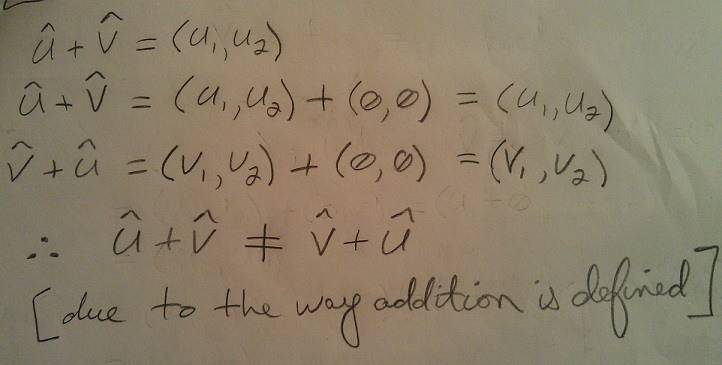not sure if this is the proper way to write something like this up. . .

It's true that this addition operation isn't commutative, but all you have done is to verify that 1) when $\vec v=(0,0)$, we have $\vec u+\vec v=\vec u+(0,0)=\vec u$, and 2) when $\vec u=(0,0)$, we have $\vec v+\vec u=\vec v+(0,0)=\vec v$. This does not prove that the addition operation is non-commutative.

What you have to do to prove that this addition operation isn't commutative is to verify that there are two vectors $\vec u,\vec v$ such that $\vec u+\vec v\neq\vec v+\vec u$.

Last edited: